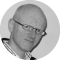Screenwriter, Choreographer, Game Developer

# PHP 5.2 - Nesting level too deep - recursive dependency?

Posted on

I installed PHP 5.2 on one of my testing servers today and a couple of bits of code that previously worked fine in version 5.1.6 threw fatal errors in the new version. The error message was "Nesting level too deep - recursive dependency?" and it took a little time to track down the root of the problem. Here's what I'd done wrong.

In PHP there are two comparison operators, `==` and `===`. It's generally known that the first is not strict about type but the second is. So, for example

```echo ( false == 0 ); // true
echo ( false === 0 ); // false
- 0 is an integer and false is a boolean```

My problem arose from using non-strict typing with objects.

```\$a = new MyObj();
\$b = new MyObj();
if( \$a == \$b )
...```

I hadn't considered what I was doing with this code. When comparing two objects using the non-strict comparison operator (==) PHP compares all the properties of the objects and if they match the objects are deemed to be equal. If they don't match they are not equal. In effect, we have a recursive comparison of all the properties of each object, and all their properties, etc. until we reach basic data types like strings and integers.

If, however, we use strict comparison (===), PHP will check whether the two objects are exactly the same object, not just objects with the same properties.

```class MyObj
{
public \$p;
}

\$a = new MyObj();
\$b = new MyObj();
\$c = new MyObj();
\$a->p = 1;
\$b->p = 1;
\$c->p = 2;
echo ( \$a == \$c ); // false
echo ( \$a == \$b ); // true
echo ( \$a === \$b ); // false```

The problem arises if you have circular references in your objects properties. So, for example

```class MyObj
{
public \$p;
}
class OtherObj
{
public \$q;
}

\$a = new MyObj();
\$b = new OtherObj();
\$a->p = \$b;
\$b->q = \$a; // the circular reference: \$a->p->q === \$a

\$c = new MyObj();
\$d = new OtherObj();
\$c->p = \$d;
\$d->q = \$c;// another circular reference: \$c->p->q === \$c

echo ( \$a == \$c ); // Fatal error:
Nesting level too deep - recursive dependency?```

In order to compare \$a to \$c, PHP must compare their properties. So the logic in PHP goes something like this: `\$a == \$c if \$a->p == \$c->p if \$a->p->q == \$c->p->q if \$a->p->q->p == \$c->p->q->p` etc. indefinitely.

PHP 5.1 seemed to smooth over the problem somehow (probably after a certain level of recursion it simply returned false) - and usually it worked out fine. PHP 5.2 correctly produces the fatal error above.

Once you know the problem, the solution is easy - use strict comparison.

`echo ( \$a === \$c ); // false (and no error)`

The strict comparison will simply check whether the two objects are at the same location in memory and so doesn't even look at the values of the properties.

N.B. The same problem can arise when using the negated comparison operators (use `!==` instead of `!=`) and when using `in_array` (use `in_array`'s third parameter to indicate strict comparison).

Share this post or a comment online -

Also in the collection PHP